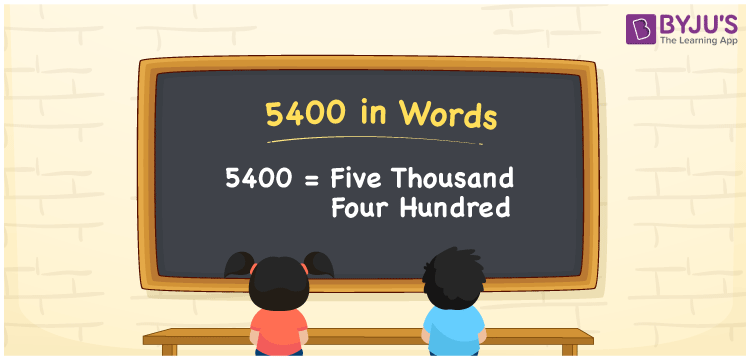# 5400 in Words

We can write 5400 in words as Five thousand four hundred. If you paid Rs. 5400 for groceries, then you can say that “I paid five thousand four hundred rupees for groceries”. That means we use the word form of numbers to convey a certain amount of money or quantities. Let’s learn how to convert the number 5400 into word form here.

 5400 in words Five thousand four hundred Five thousand four hundred in Numbers 5400

## 5400 in English Words

We generally write numbers in words with the help of the English alphabet. Thus, we can express 5400 in English words as “Five thousand four hundred”.## How to Write 5400 in Words?

We know that 5400 is a four-digit number so let’s make a place value chart with four place values in four columns as shown below.

 Thousands Hundreds Tens Ones 5 4 0 0

Here, ones = 0, tens = 0, hundreds = 4, and thousands = 5.

By expanding these numbers, we get;

5 × Thousand + 4 × Hundred + 0 × Ten + 0 × One

= 5 × 1000 + 4 × 100 + 0 × 10 + 0 × 1

= 5000 + 400

= Five thousand + Four hundred

= Five thousand four hundred

Therefore, 5400 in words = Five thousand four hundred.

Also, check: place value

5400 is a natural number that precedes 5401 and succeeds 5399.

5400 in words – Five thousand four hundred

Is 5400 an odd number? – No

Is 5400 an even number? – Yes

Is 5400 a prime number? – No

Is 5400 a composite number? – Yes

Is 5400 a perfect square number? – No

Is 5400 a perfect cube number? – No

## Frequently Asked Questions on 5400 in Words

Q1

### How do you write 5400 in words?

We can write the number 5400 in words as Five thousand four hundred.
Q2

### How to write Rs. 5400 in words on a cheque?

On a cheque, we generally write Rs. 5400 in words as Five thousand four hundred rupees only.
Q3

### What number is five thousand four hundred in word form?

The number 5400 is five thousand four hundred, i.e. the word form of 5400 is five thousand four hundred.